# Summarize Within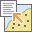The Summarize Within tool calculates statistics in areas where an input layer overlaps a boundary layer.

## Workflow diagram## Examples

• A city is trying to deal with a backlog of construction projects and wants to involve the maintenance assessment districts in projects related to streetlight and bike route maintenance. The Summarize Within tool is used to count the number of streetlights and miles of bike routes in each district so a subset of districts can be selected as a starting point.

• A cable provider is starting a pilot program where it provides low-cost Internet access to low-income community college students. Summarize Within can be used to determine the number of low-income families in each college district so the cable provider can choose an appropriate district for its pilot program.

• A development company is looking to make some money by creating a new mixed-use development in an urban center. The development will have to be conveniently located within a quarter mile of shops, restaurants, or a light rail stop. The qualifying areas can be summarized within the city's boundary to find the total area.

## Usage notes

The inputs for Summarize Within include one area layer that serves as the boundary for summarizing features and a point, line, or area layer to be summarized.

##### Tip:

Depending on the configuration of your organization, you will have access to either Esri Living Atlas Analysis Layers, such as counties and hexbins, or Custom Analysis Layers. Click the drop-down arrow for the Choose area layer to summarize other features within its boundaries parameter to select an analysis layer to use as a boundary.

A Count of Points, Total Length, or Total Area box will appear depending on the type of features to summarize in your layer. The boxes are checked by default and can only be unchecked if statistics are being calculated. The default distance measure will depend on the Units in your profile.

TotalInput FeaturesDefaultOptions

Count of Points

Points

None

None

Total Length

Lines

Miles (U.S. Standard setting) or Kilometers (Metric setting)

• Miles
• Feet
• Kilometers
• Meters
• Yards

Total Area

Areas

Square Miles (U.S. Standard setting) or Square Kilometers (Metric setting)

• Square Miles
• Square Kilometers
• Square Meters
• Hectares
• Acres

There are five options for statistics that can be calculated on numeric fields in the layer to be summarized: sum, minimum, maximum, average, and standard deviation. Each time a Field and Statistic is entered, a new row will be added to the tool pane so more than one statistic can be calculated at once. The summarized data can be viewed in the result layer's table or pop-ups.

Optionally, a group by field can be selected so statistics are calculated separately for each unique attribute value. When a group by field is selected, the pop-up for each of the features in the output layer will contain charts showing each summary count or total and statistic by field value. A summary table listing each feature and statistic by group by field value will also be created.

The Add minority, majority and Add percentages boxes are enabled when a group by field is entered. The minority and majority will be the least and most dominant value from the group by field, respectively, where dominance is determined using the count of points, total length, or total area of each value. When Add minority, majority is checked, two new fields will be added to the result layer. The fields will list the values from the group field that are the minority and majority for each result feature. When Add percentages is checked, two new fields will be added to the result layer listing the percentage of the count of points, total length, or total area that belong to the minority and majority values for each feature. A percentage field will also be added to the result table listing the percentage of the count of points, total length, or total area that belong to all values from the group by field for each feature.

If Use current map extent is checked, only those features in the input layer and the layer to be summarized that are visible within the current map extent will be analyzed. If unchecked, all features in both the input layer and the layer to be summarized will be analyzed, even if they are outside the current map extent.

## Limitations

• The boundary input must be area features.
• Lines and areas are summarized using proportions; therefore, it is best to summarize absolute data (such as population) rather than relative data (such as average income) when lines or areas are being summarized.
• There is no option to remove boundaries that do not overlap any point, lines, or areas.

## How Summarize Within works

### Equations

Average and Std Deviation are calculated using weighted mean and weighted standard deviation for line and area features. None of the statistics for point features are weighted. The following table shows the equations used to calculate standard deviation, weighted mean, and weighted standard deviation.

StatisticEquationVariablesFeatures

Standard Deviation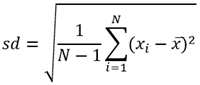Points

Weighted Mean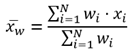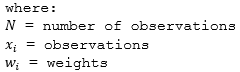Lines and Areas

Weighted Standard Deviation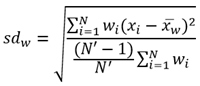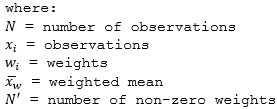Lines and Areas

### Points

Point layers are summarized using only the point features within the input boundary. The results will be displayed using graduated symbols.

The figure and table below explain the statistical calculations of a point layer within a hypothetical boundary. The Population field was used to calculate the statistics (Sum, Minimum, Maximum, Average, and Std Deviation) for the layer.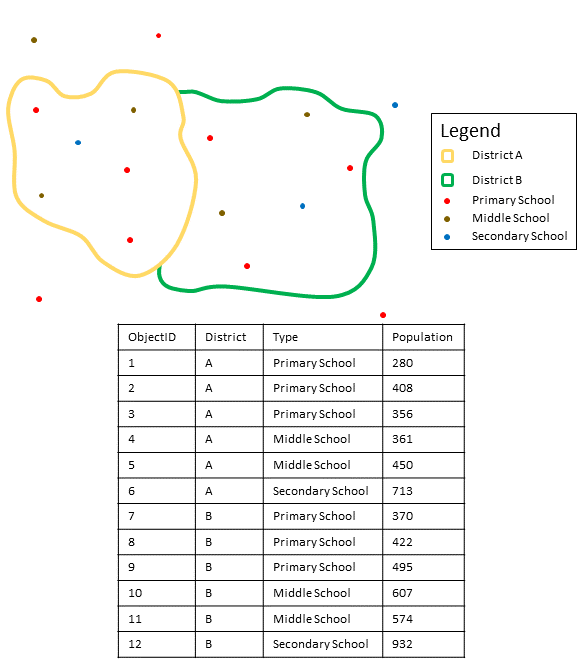Point layers are summarized using only points located within the boundary layer. An example attribute table is displayed above with values to be used in hypothetical statistic calculations.
StatisticResults District A

Sum

``280 + 408 + 356 + 361 + 450 + 713 = 2,568``

Minimum

Minimum of:

``[280, 408, 356, 361, 450, 713] = 280``

Maximum

Maximum of:

``[280, 408, 356, 361, 450, 713] = 713``

Average

``2,568/6 = 428``

Std Deviation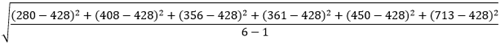``= 150.79``

A real-life scenario in which this analysis could be used is in determining the total number of students in each school district. Each point represents a school. The Type field gives the type of school (elementary, middle school, or secondary) and a student population field gives the number of students enrolled at each school. The calculations and results are given for District A in the table above. From the results you can see that District A has 2,568 students. When running the Summarize Within tool, the results would also be given for District B.

### Lines

Line layers are summarized using only the proportions of the line features that are within the input boundary. When summarizing lines, use fields with absolute numbers so proportional calculations make logical sense in your analysis. The results will be displayed using graduated symbols.

The figure and table below explain the statistical calculations of a line layer within a hypothetical boundary. The Volume field was used to calculate the statistics (Sum, Minimum, Maximum, Average, and Std Deviation) for the layer. The statistics are calculated using only the proportion of the lines that are within the boundary.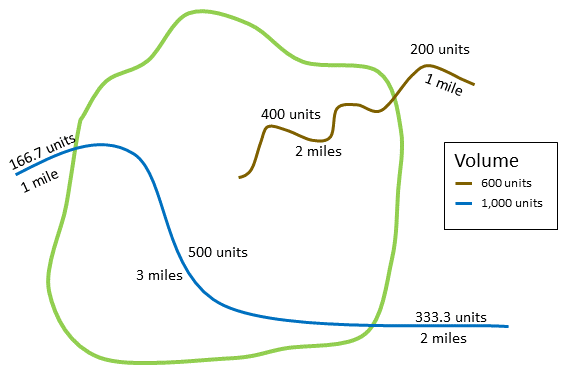Line layers are summarized using only the proportions of the lines located within the boundary.
StatisticResult

Sum

``400 + 500 = 900``

Minimum

Minimum of:

``[400, 500] = 400``

Maximum

Maximum of:

``[400, 500] = 500``

Average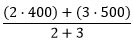``= 460``

Std Deviation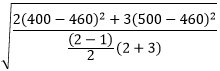``= 69.28``

A real-life scenario in which this analysis could be used is in determining the total volume of water in rivers within the boundaries of a state park. Each line represents a river that is partially located inside the park. From the results, you can see that there are 5 miles of rivers within the park and the total volume is 900 units.

### Areas

Area layers are summarized using only the proportions of the area features that are within the input boundary. When summarizing areas, use fields with absolute numbers so proportional calculations make logical sense in your analysis. The results layer will be displayed using graduated colors.

The figure and table below explain the statistical calculations of an area layer within a hypothetical boundary. The populations were used to calculate the statistics (Sum, Minimum, Maximum, Average, and Std Deviation) for the layer. The statistics are calculated using only the proportion of the area that is within the boundary.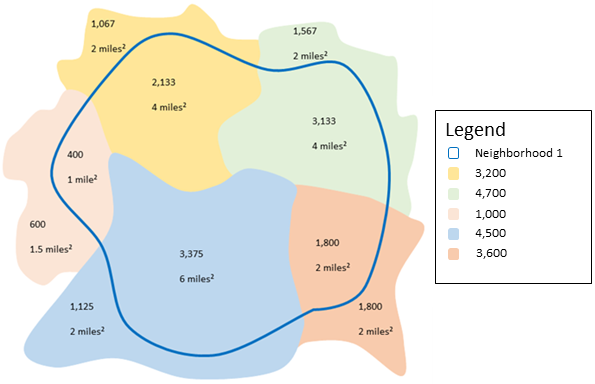Area layers are summarized using only the proportions of the areas located within the boundary.
StatisticResult

Sum

``2,133 + 3,133 + 400 + 3,375 + 1,800 = 10,841``

Minimum

Minimum of:

``[2,133, 3,133, 400, 3,375, 1,800] = 400``

Maximum

Maximum of:

``[2,133, 3,133, 400, 3,375, 1,800] = 3,375``

Average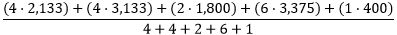``= 2,665.53``

Std Deviation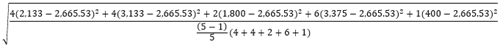``= 925.91``

A real-life scenario in which this analysis could be used is in determining the population in a city neighborhood. The blue outline represents the boundary of the neighborhood and the smaller areas represent census blocks. From the results, you can see that there are 10,841 people in the neighborhood and an average of approximately 2,666 people per census block.

## Similar tools

Use Summarize Within to calculate statistics for features that overlap a boundary layer. Other tools may be useful in solving similar but slightly different problems.

### Map viewer analysis tools

If you are trying to summarize points and only want to keep the boundaries with a count greater than zero, use the Aggregate Points tool.

If you are trying to summarize features within a distance of your input features, use the Summarize Nearby tool.

### ArcGIS Desktop analysis tools

Summarize Within performs the functions of the Spatial Join and Summary Statistics tools.

Summarize Within is also available in ArcGIS Pro. To run the tool from ArcGIS Pro, your project's active portal must be running Portal for ArcGIS 10.5 or later. You must also sign in to the portal using an account that has privileges to perform standard feature analysis in the portal.